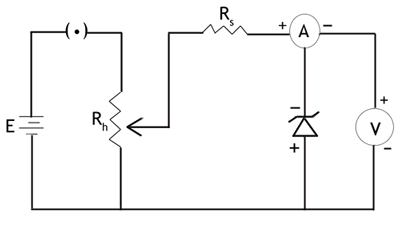you are here->home->Physics->Class 12->Zener Diode
Zener Diode

# Materials required:

• Battery
• Rheostat
• Small resistance (200 Ω)
• Milliammeter
• Voltmeter
• Key
• Zener diode

# Real Lab Procedure:

• Connections are made as shown in the circuit diagram.• By adjusting the rheostat, voltmeter reading is increased from 0 and in each time note the corresponding reading in milliammeter.
• The experiment is continued till the milliammeter shows a large deflection while the voltmeter reading remains a constant, indicating the break down voltage.
• Plot the reverse characteristic curve by taking reverse voltage along –ve X-axis and reverse current along –ve Y-axis.
• The break down voltage Vz is obtained from the graph as shown below.

# Simulator Procedure:

• Select the Zener diode from the drop down list.
• To see the circuit diagram, click on the 'Show/hide circuit diagram' check box seen inside the simulator window.
• Connections can be made as seen in the circuit diagram by clicking and dragging the mouse from one connecting terminal to the other connecting terminal of the devices to be connected.
• Drag the key to insert it.
• You can change the rheostat resistance using the slider.
• You can notice the corresponding ammeter and voltmeter readings.
• You can use the embedded worksheet to enter the values.
• To see the graph, click on the graph icon in the worksheet.
• To redo the experiment, click on the ‘Reset’ button.

# Observations:

Least count of voltmeter = ...........V

Zero error of voltmeter  = ............V

Least count of milli-ammeter  = ...........mA

Zero error of milli-ammeter = ...........mA

 Trial No. Reverse Voltage (V) Reverse Current (mA) 1 2 3 4 5 6 7 8 9 10

# Calculation:

Plot the reverse characteristic curve by taking reverse voltage along –ve X-axis and reverse current along –ve Y-axis.

# Result:

• The reverse characteristic curve of the Zener diode is obtained.
• The reverse breakdown voltage of the Zener diode, V = ………….V